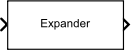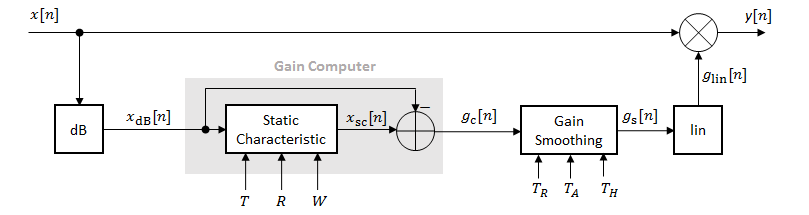# Expander

Dynamic range expander

• Library:
• Audio Toolbox / Dynamic Range Control## Description

The Expander block performs dynamic range expansion independently across each input channel. Dynamic range expansion attenuates the volume of quiet sounds below a given threshold. The block uses specified attack, release, and hold times to achieve a smooth applied gain curve.

## Ports

### Input

expand all

• Matrix input –– Each column of the input is treated as an independent channel.

• 1-D vector input –– The input is treated as a single channel.

This port is unnamed unless you specify additional input ports.

Data Types: `single` | `double`

#### Dependencies

To enable this port, select Specify from input port for the Ratio parameter.

Data Types: `single` | `double`

#### Dependencies

To enable this port, select Specify from input port for the Threshold (dB) parameter.

Data Types: `single` | `double`

#### Dependencies

To enable this port, select Specify from input port for the Knee width (dB) parameter.

Data Types: `single` | `double`

#### Dependencies

To enable this port, select Specify from input port for the Attack time (s) parameter.

Data Types: `single` | `double`

#### Dependencies

To enable this port, select Specify from input port for the Release time (s) parameter.

Data Types: `single` | `double`

#### Dependencies

To enable this port, select Specify from input port for the Hold time (s) parameter.

Data Types: `single` | `double`

### Output

expand all

The Expander block outputs a signal with the same data type as the input signal. The size of the output depends on the size of the input:

• Matrix input –– The block outputs a matrix the same size and data type as the input signal.

• 1-D vector input –– The block outputs an N-by-1 matrix (column vector), where N is the number of elements in the 1-D vector.

This port is unnamed until you select the Output gain (dB) parameter.

Data Types: `single` | `double`

#### Dependencies

To enable this port, select the Output gain (dB) parameter.

Data Types: `single` | `double`

## Parameters

expand all

If a parameter is listed as tunable, then you can change its value during simulation.

#### Main Tab

Expansion ratio is the input/output ratio for signals that undershoot the operation threshold.

Assuming a hard knee characteristic and a steady-state input such that x[n] dB < Threshold (dB), the expansion ratio is defined as $R=\frac{\left(y\left[n\right]-T\right)}{\left(x\left[n\right]-T\right)}$, where

• R is the expansion ratio.

• y[n] is the output signal in dB.

• x[n] is the input signal in dB.

• T is the threshold in dB.

To specify Ratio from an input port, select Specify from input port for the parameter.

Tunable: Yes

Operation threshold is the level below which gain is applied to the input signal.

To specify Threshold (dB) from an input port, select Specify from input port for the parameter.

Tunable: Yes

For soft knee characteristics, the transition area is defined by the relation

`$y=x+\frac{\left(1-R\right)×{\left(x-T-\frac{W}{2}\right)}^{2}}{\left(2×W\right)}$`

for the range $\left(2×|x-T|\right)\le W$, where

• y is the output level in dB.

• x is the input level in dB.

• R is the expansion ratio.

• T is the threshold in dB.

• W is the knee width in dB.

To specify Knee width (dB) from an input port, select Specify from input port for the parameter.

Tunable: Yes

The plot is updated automatically when parameters of the Expander block change.

Tunable: Yes

Attack time is the time the expander gain takes to rise from 10% to 90% of its final value when the input goes below the threshold. The Attack time (s) parameter smooths the applied gain curve.

To specify Attack time (s) from an input port, select Specify from input port for the parameter.

Tunable: Yes

Release time is the time the expander gain takes to drop from 90% to 10% of its final value when the input goes above the threshold. The Release time (s) parameter smooths the applied gain curve.

To specify Release time (s) from an input port, select Specify from input port for the parameter.

Tunable: Yes

Hold time is the period in which the applied gain is held constant before it starts moving toward its steady-state value. Hold time begins when the input level crosses the operation threshold.

To specify Hold time (s) from an input port, select Specify from input port for the parameter.

Tunable: Yes

When you select this parameter, the block inherits its sample rate from the input signal. When you clear this parameter, specify the sample rate in the Input sample rate (Hz) parameter.

Tunable: No

Tunable: Yes

#### Dependencies

To enable this parameter, clear the Inherit sample rate from input parameter.

When you select this parameter, an additional output port, G, is added to the block. The G port outputs the gain applied on each input channel in dB.

Tunable: No

• `Interpreted execution` –– Simulate model using the MATLAB® interpreter. This option shortens startup time and has a simulation speed comparable to ```Code generation```. In this mode, you can debug the source code of the block.

• `Code generation` –– Simulate model using generated C code. The first time you run a simulation, Simulink® generates C code for the block. The C code is reused for subsequent simulations, as long as the model does not change. This option requires additional startup time, but the speed of the subsequent simulations is comparable to ```Interpreted execution```.

Tunable: No

## Block Characteristics

 Data Types `double` | `single` Direct Feedthrough `no` Multidimensional Signals `no` Variable-Size Signals `yes` Zero-Crossing Detection `no`

## Algorithms

The Expander block processes a signal frame by frame and element by element.1. The N-point signal, x[n], is converted to decibels:

`${x}_{\text{dB}}\left[n\right]=20×{\mathrm{log}}_{10}|x\left[n\right]|$`

2. xdB[n] passes through the gain computer. The gain computer uses the static characteristic properties of the dynamic range expander to attenuate gain that is below the threshold.

If you specified a soft knee, the gain computer has the following static characteristic:

`${x}_{\text{sc}}\left({x}_{\text{dB}}\right)=\left\{\begin{array}{cc}T+\left({x}_{\text{dB}}-T\right)×R& {x}_{\text{dB}}<\left(T-\frac{W}{2}\right)\\ {x}_{\text{dB}}+\frac{\left(1-R\right){\left({x}_{\text{dB}}-T-\frac{W}{2}\right)}^{2}}{2W}& \left(T-\frac{W}{2}\right)\le {x}_{\text{dB}}\le \left(T+\frac{W}{2}\right)\\ {x}_{\text{dB}}& {x}_{\text{dB}}>\left(T+\frac{W}{2}\right)\end{array}\text{ },$`

where T is the threshold, R is the expansion ratio, and W is the knee width.

If you specified a hard knee, the gain computer has the following static characteristic:

`${x}_{\text{sc}}\left({x}_{\text{dB}}\right)=\left\{\begin{array}{cc}T+\left({x}_{\text{dB}}-T\right)×R& {x}_{\text{dB}}`

3. The computed gain, gc[n], is calculated as

`${g}_{\text{c}}\left[n\right]={x}_{\text{sc}}\left[n\right]-{x}_{\text{dB}}\left[n\right].$`

4. gc[n] is smoothed using specified attack, release, and hold time parameters:

`${g}_{\text{s}}\left[n\right]=\left\{\begin{array}{c}{\alpha }_{\text{A}}{g}_{\text{s}}\left[n-1\right]+\left(1-{\alpha }_{\text{A}}\right){g}_{\text{c}}\left[n\right]\\ {g}_{\text{s}}\left[n-1\right]\text{\hspace{0.17em}}\\ {\alpha }_{\text{R}}{g}_{\text{s}}\left[n-1\right]+\left(1-{\alpha }_{\text{R}}\right){g}_{\text{c}}\left[n\right]\text{ }\\ {g}_{\text{s}}\left[n-1\right]\end{array}\text{ }\begin{array}{c}\left({C}_{\text{A}}>{T}_{\text{H}}\right)\text{\hspace{0.17em}}\text{\hspace{0.17em}}&\text{\hspace{0.17em}}\text{\hspace{0.17em}}\left({g}_{\text{c}}\left[n\right]\le {g}_{\text{s}}\left[n-1\right]\right)\\ {C}_{\text{A}}\le {T}_{\text{H}}\\ \left({C}_{\text{R}}>{T}_{\text{H}}\right)\text{\hspace{0.17em}}\text{\hspace{0.17em}}&\text{\hspace{0.17em}}\text{\hspace{0.17em}}\left({g}_{\text{c}}\left[n\right]>{g}_{\text{s}}\left[n-1\right]\right)\\ {C}_{\text{R}}\le {T}_{\text{H}}\text{\hspace{0.17em}}\end{array}$`

CA and CR are hold counters for attack and release, respectively. The limit, TH, is determined by the Hold time (s) parameter.

The attack time coefficient, α A, is calculated as

`${\alpha }_{\text{A}}=\mathrm{exp}\left(\frac{-\mathrm{log}\left(9\right)}{Fs×{T}_{\text{A}}}\right)\text{\hspace{0.17em}}.$`

The release time coefficient, α R, is calculated as

`${\alpha }_{\text{R}}=\mathrm{exp}\left(\frac{-\mathrm{log}\left(9\right)}{Fs×{T}_{\text{R}}}\right)\text{\hspace{0.17em}}.$`

TA is the attack time period, specified by the Attack time (s) parameter. T R is the release time period, specified by the Release time (s) parameter. Fs is the input sampling rate, specified by the Inherit sample rate from input or Input sample rate (Hz) parameter.

5. The smoothed gain in dB, gs[n], is translated to a linear domain:

`${g}_{\text{lin}}\left[n\right]={10}^{\left(\frac{{g}_{\text{s}}\left[n\right]}{20}\right)}\text{ }.$`

6. The output of the dynamic range expander is given as

`$y\left[n\right]=x\left[n\right]×{g}_{\text{lin}}\left[n\right].$`

 Giannoulis, Dimitrios, Michael Massberg, and Joshua D. Reiss. "Digital Dynamic Range Compressor Design –– A Tutorial And Analysis." Journal of Audio Engineering Society. Vol. 60, Issue 6, 2012, pp. 399–408.

##### Support